﻿ 基于ZigBee技术的空间电荷密度测量系统
«上一篇文章快速检索 高级检索

 应用科技2017, Vol. 44Issue (3): 19-24  DOI: 10.11991/yykj.2016050100

### 引用本文SUN Dingyong, YUAN Haiwen, LYU Jianxun, et al. Space charge density measurement system based on ZigBee technology[J]. Applied Science and Technology, 2017, 44(3), 19-24. DOI: 10.11991/yykj.201605010.### 文章历史

1. 北京航空航天大学 自动化科学与电气工程学院, 北京 100191;
2. 中国电力科学研究院, 北京 100192

Space charge density measurement system based on ZigBee technology
SUN Dingyong1, YUAN Haiwen1, LYU Jianxun1, ZHANG Yuekui1, JU Yong2, YANG Xiaohong2
1. School of Automation Science and Electrical Engineering, Beihang University, Beijing 100191, China;
2. China Electric Power Science Research Institute, Beijing 100192, China
Abstract: In order to measure the space charge density in the ultra high voltage direct current (UHVDC) transmission line, a distributed measurement system was designed and implemented.Measurement system has a number of space charge density measurement devices, besides the measurement of the device was based on the principle of the ion counter method.The coaxial cylinder type electrode plate was used as the charge density sensor, and the axial flow fan and the flow meter were assembled on the sensor.The device adopted the STM32 chip as the core controller, and communicated with the host computer through the mode of the ZigBee wireless transmission.The lower computer software was developed with the C language, and the upper computer software with the C#WinForm program.Multi-thread concurrent technology was used in the development of the software.And the measurement system was used in the UHV test base in Changping, verifying the availability of the system.
Key words: space charge density    icon counter method    ZigBee    multi-thread concurrent    UHVDC    distributed measurement system    ion counter

1 测量系统的总体设计方案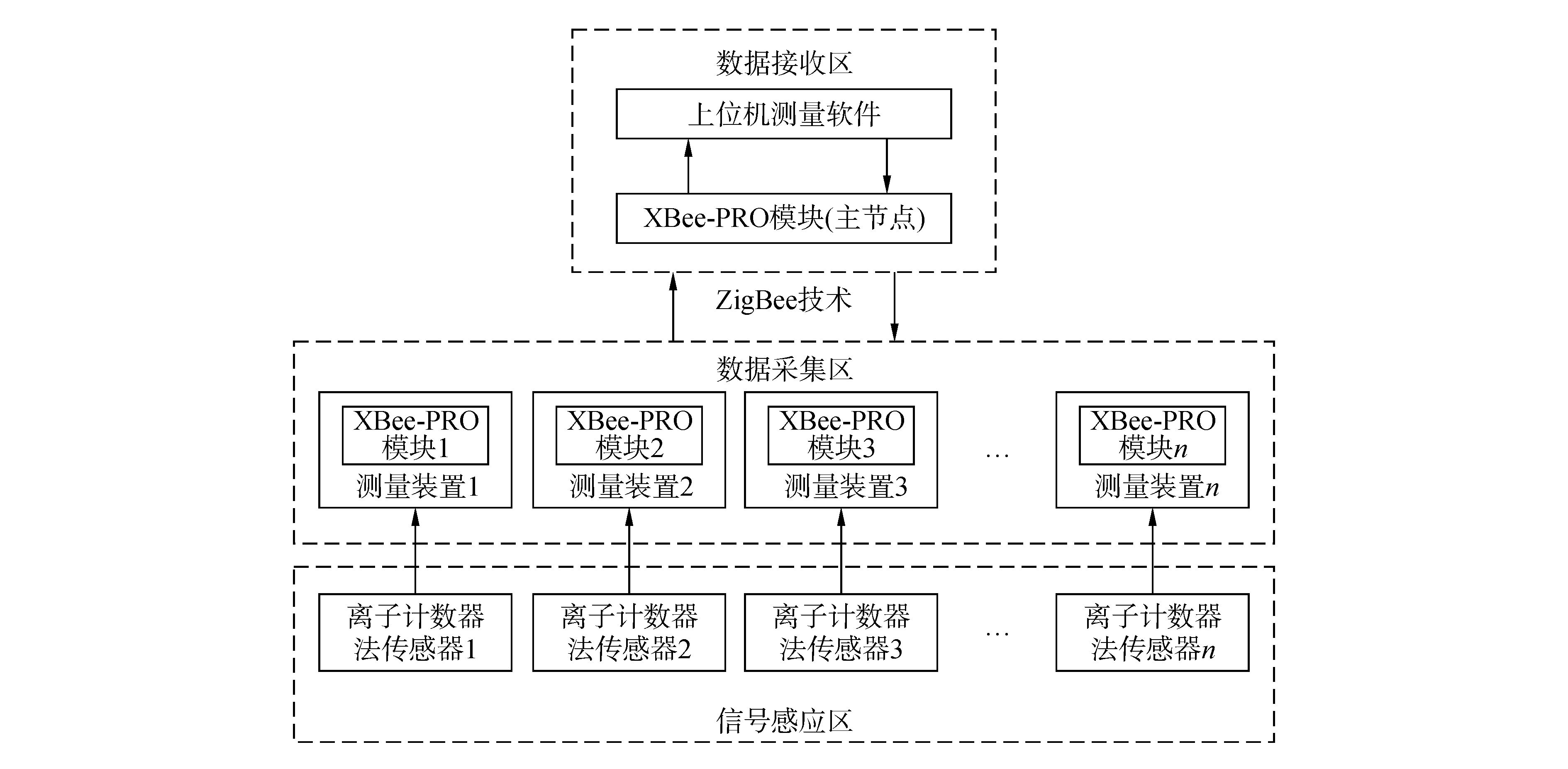图 1 测量系统的总体框架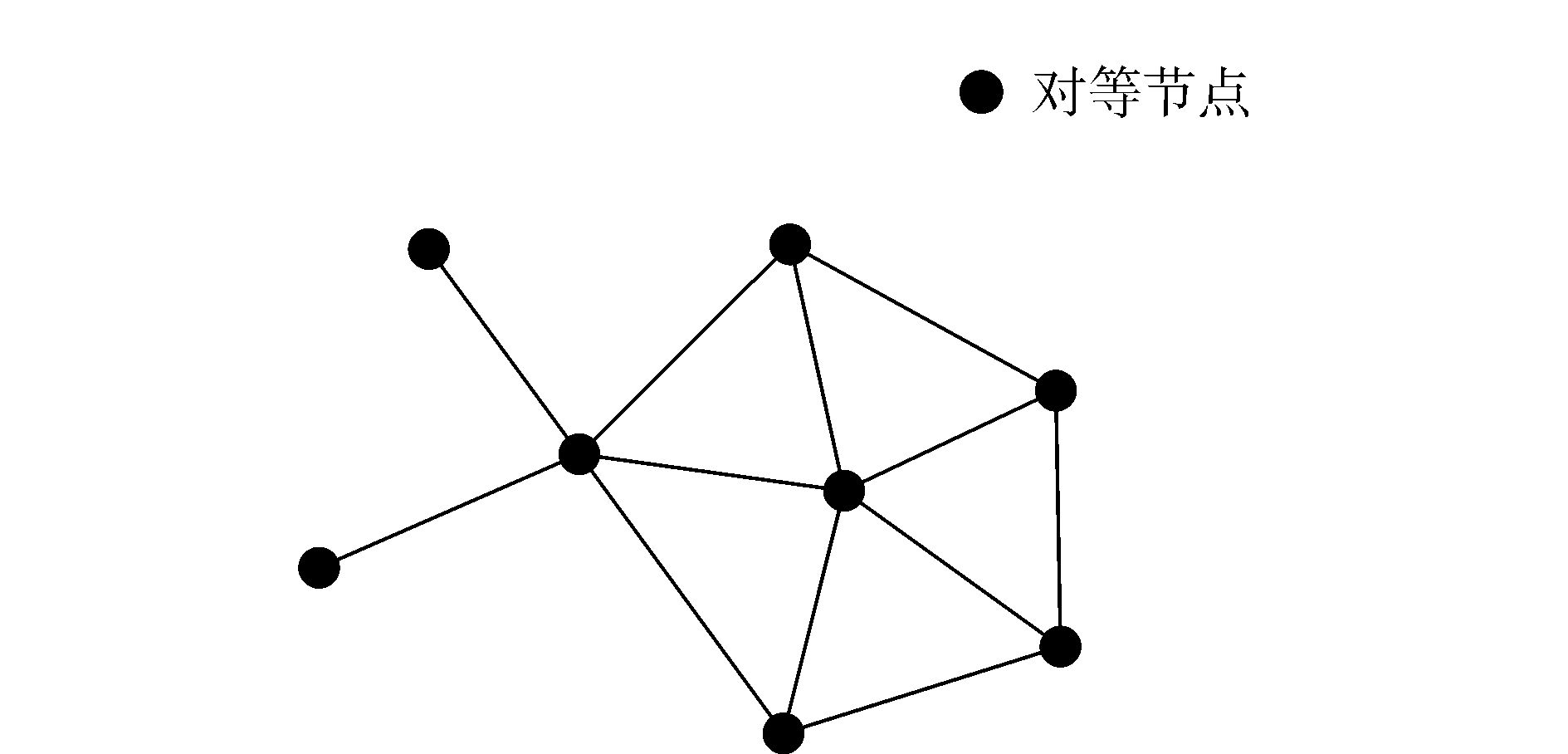图 2 DigiMesh的网络拓扑结构

2 测量系统的硬件设计

2.1 离子计数器法传感器的硬件设计 2.1.1 离子计数器法测量原理

 $\rho = \frac{Q}{V} = \frac{{\int_{{t_0}}^t {I\left( t \right){\rm{d}}t} }}{{\int_{{t_0}}^t {{\rm{ \mathsf{ π} }}({R^2} - {r^2})v\left( t \right){\rm{d}}t} }}$ (1)

2.1.2 离子计数器法传感器的设计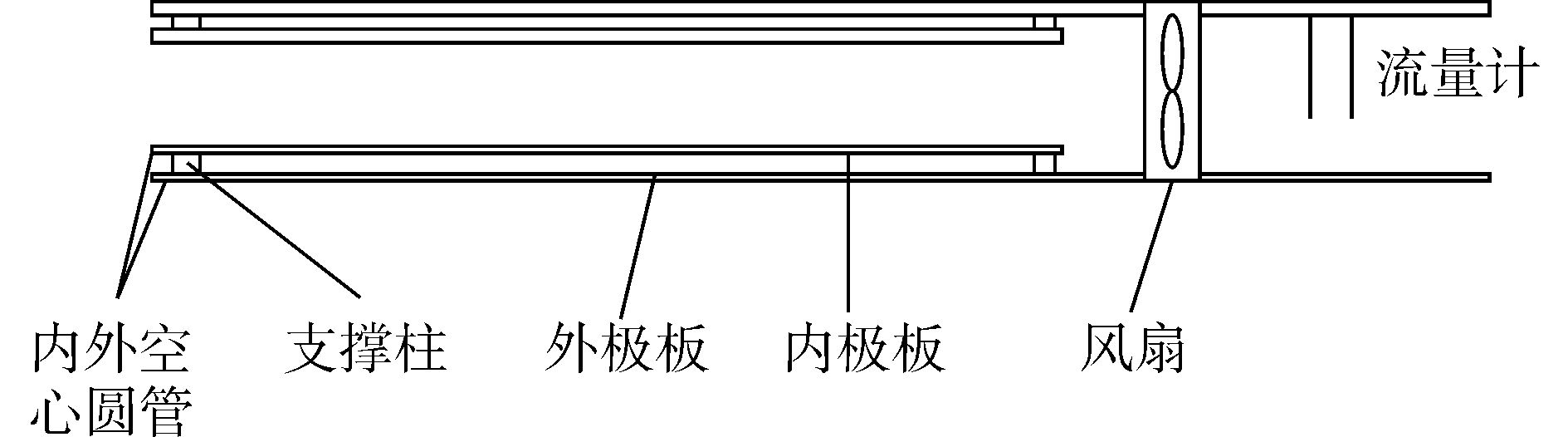图 3 离子计数器法传感器的结构表 1 Pt20与Pt300的阻值及温度特性参数表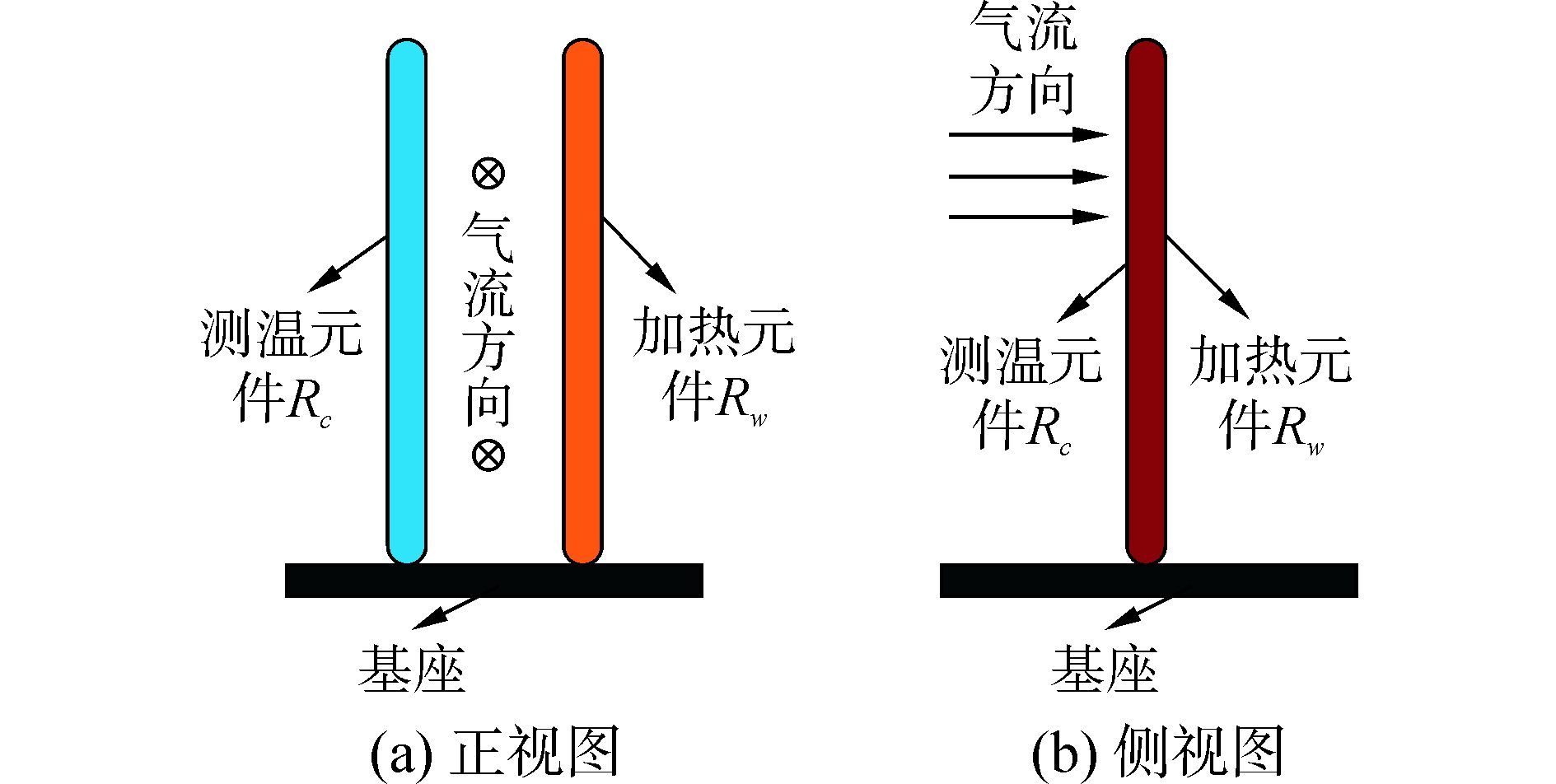图 4 流量计测量原理

 $R\left( t \right) = \left\{ \begin{array}{l} {R_0}\left[ {1 + At + B{t^2} + C\left( {t - 100} \right){t^3}} \right],\\ \quad \quad \quad \quad \quad - 200℃ ＜ t ＜ 0℃\\ {R_0}(1 + At + B{t^2}),{\rm{ }}0℃ ＜ t ＜ 850℃ \end{array} \right.$ (2)

 $H = I_w^2{R_w} = hA({T_w} - {T_c}) = h{\rm{ \mathsf{ π} }}ld({T_w} - {T_c})$ (3)

 $h = \frac{{Nu{\lambda _f}}}{d}$ (4)

 $v = 0.466\frac{\eta }{{\rho d}}\sqrt {\frac{{I_w^2}}{{0.606{\lambda _f}{\rm{\pi }}l}}\frac{{{R_w}}}{{{T_w} - {T_c}}}}$ (5)

2.2 测量装置的硬件设计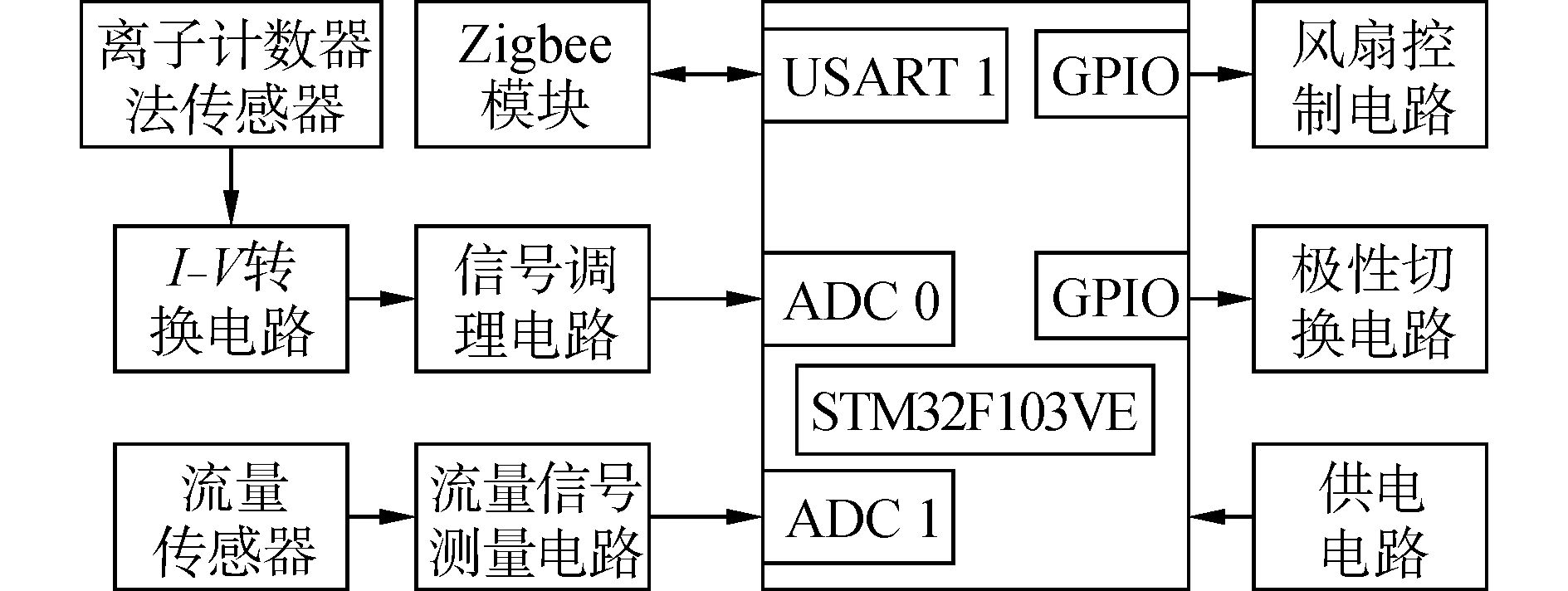图 5 测量装置的硬件结构

Xbee-Pro模块中集成了一个UART接口，发送数据经DI数据缓冲器、RF发送缓冲器、发送器等通过天线发送出去；接收数据首先被天线接收，经接收器、RF接收缓冲器、DO缓冲器后送入主机中。Xbee-Pro模块接口简单实用，可通过UART接口直接与核心处理器ARM系统的UART接口连接进行数据传输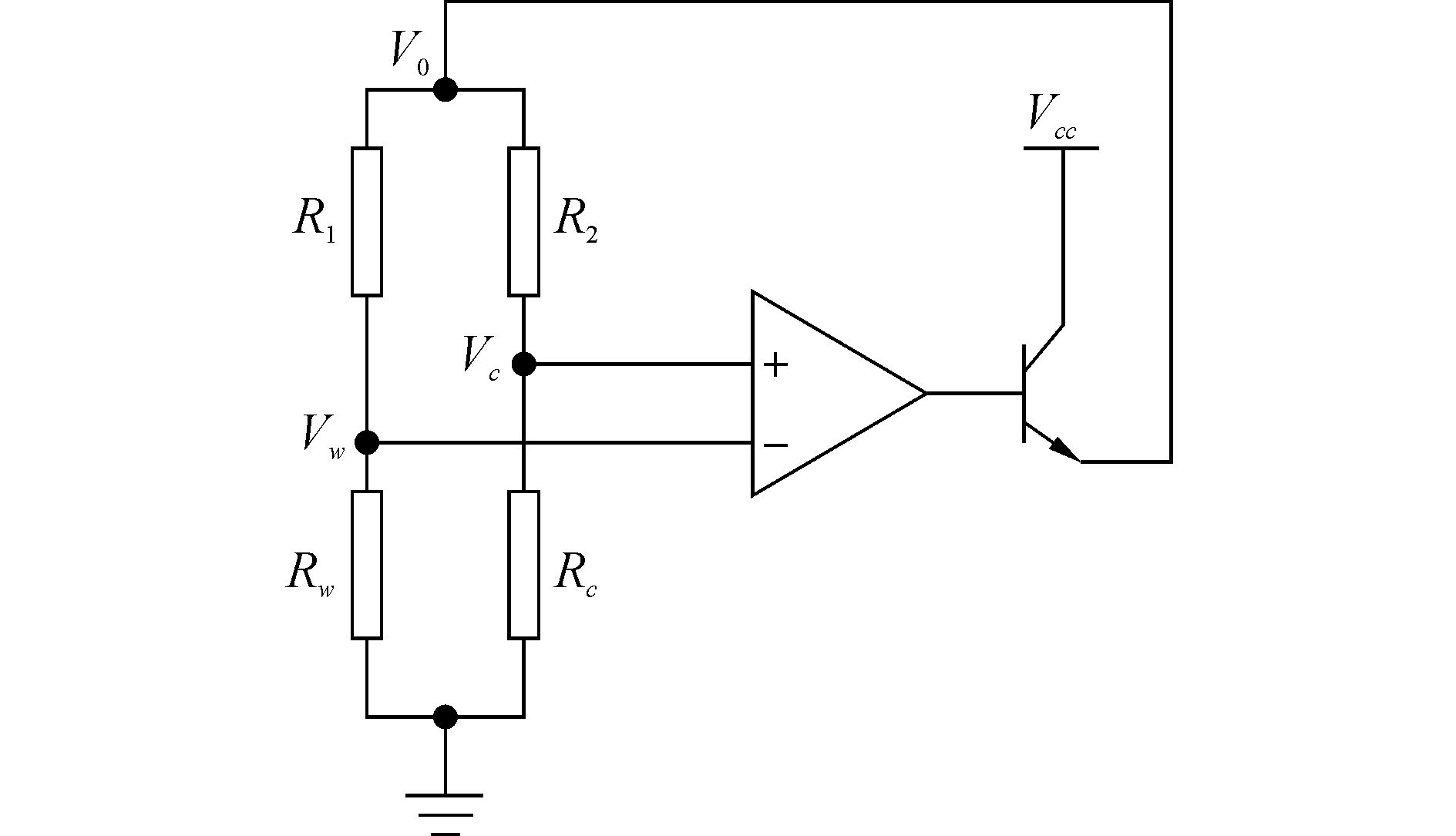图 6 流量测量电路简化模型

VwVc不相等时，此桥路的不平衡电压经过放大器后调节拱桥电压Vo。在实际电路中，本文根据所选择的热式流量传感器的参数，取R1=20 Ω，R2=300 Ω，可满足驱动电路的平衡；放大器选用LM224，供电电压为5 V，满足电路设计需求；三极管使用直插式三极管BD243C；流量计的2个测温元件均有3根引出线，2个元件均有两线并联接地，另一根与所对应的桥式电阻相连。通过测量加热元件两端电压Vw、测温元件两端电压Vc及运放输出电压Vo的值，可按照式(6)、(7) 计算出IwRwTwTc，进而求得风速。

 ${I_w} = \frac{{{V_0} - {V_w}}}{{{R_1}}},{\rm{ }}{R_w} = \frac{{{V_m}}}{{{I_m}}},{\rm{ }}{T_w} = (\frac{1}{{{A_w}}}\frac{{{R_w}}}{{{R_{{w_0}}}}} - 1)$ (6)
 ${I_c} = \frac{{{V_0} - {V_c}}}{{{R_2}}},{\rm{ }}{R_c} = \frac{{{V_c}}}{{{I_c}}},{\rm{ }}{T_c} = (\frac{1}{{{A_c}}}\frac{{{R_c}}}{{{R_{{c_0}}}}} - 1)$ (7)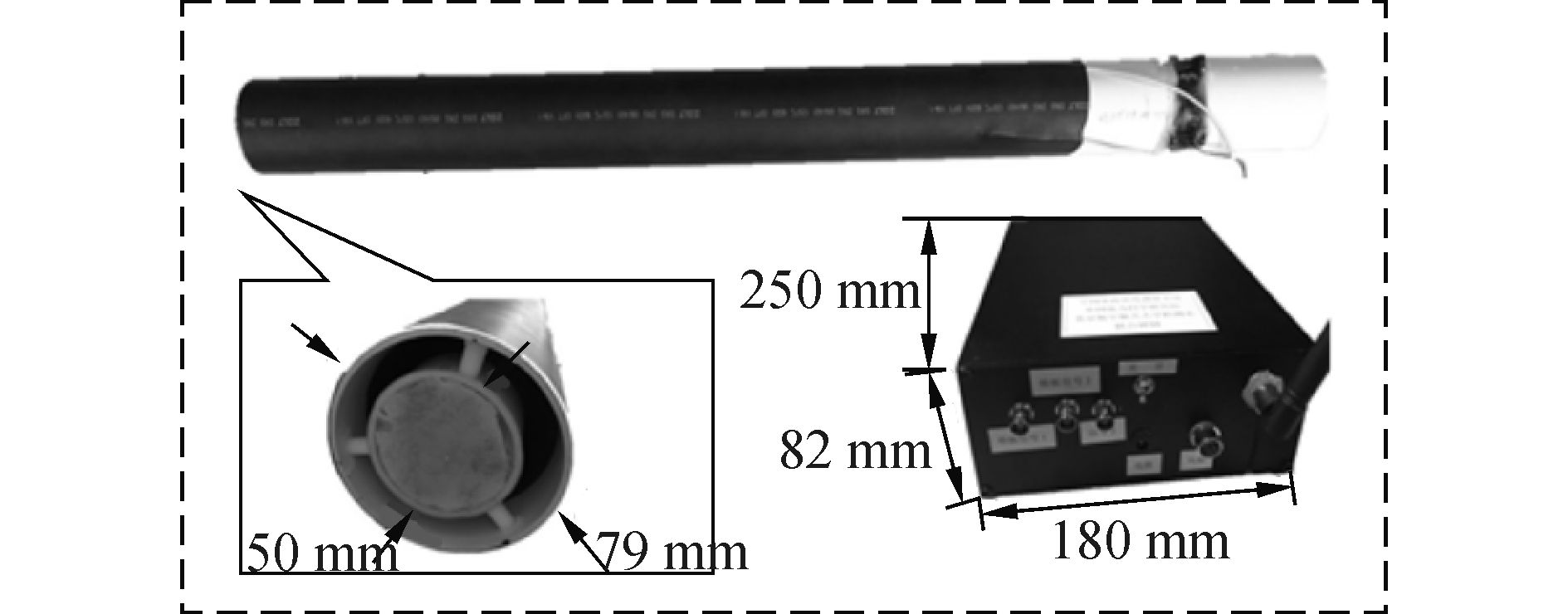图 7 离子计数器法传感器和测量装置实物

3 测量系统的软件设计 3.1 下位机软件的设计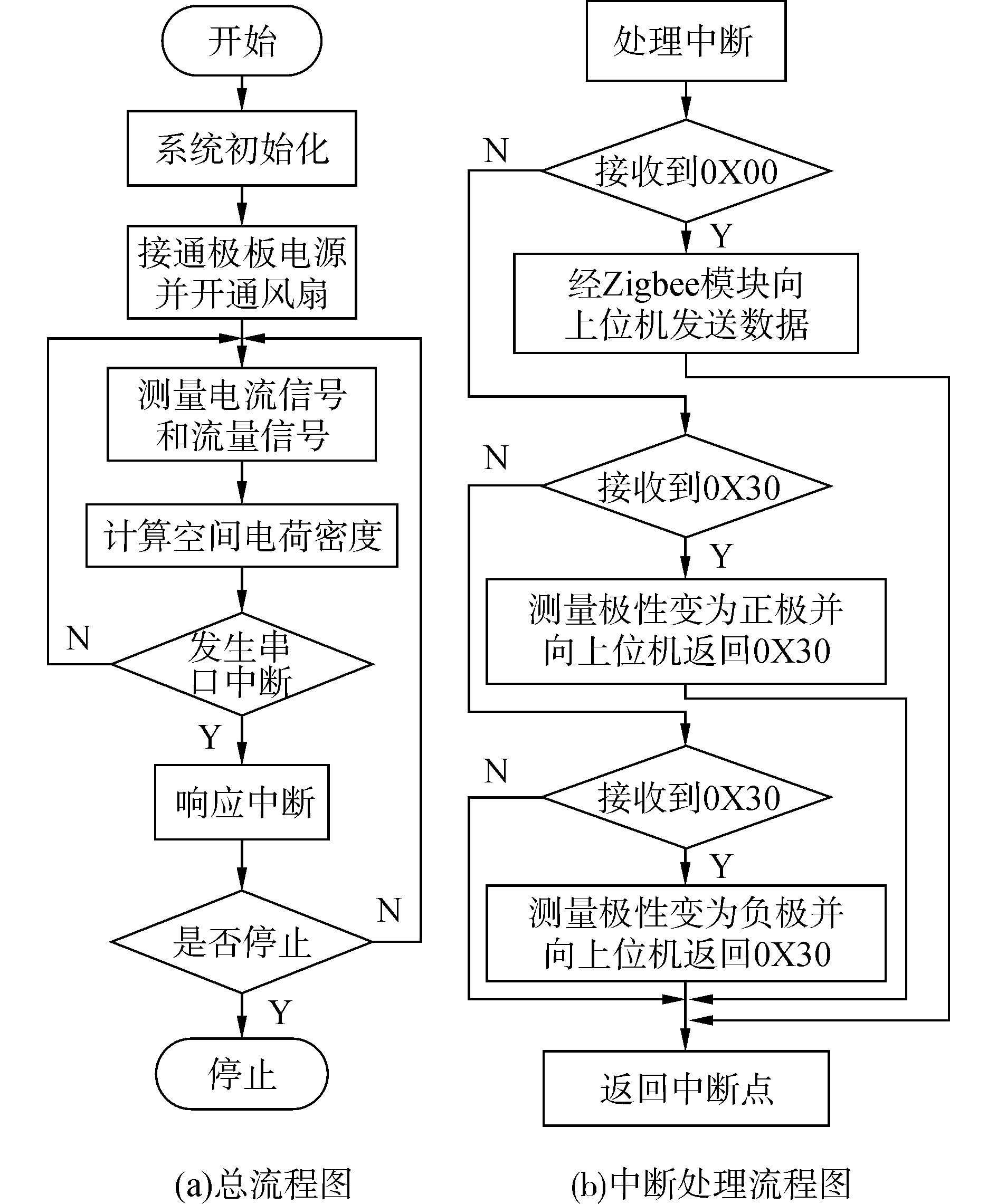图 8 下位机软件设计流程

3.2 上位机软件的设计 3.2.1 上位机软件总体结构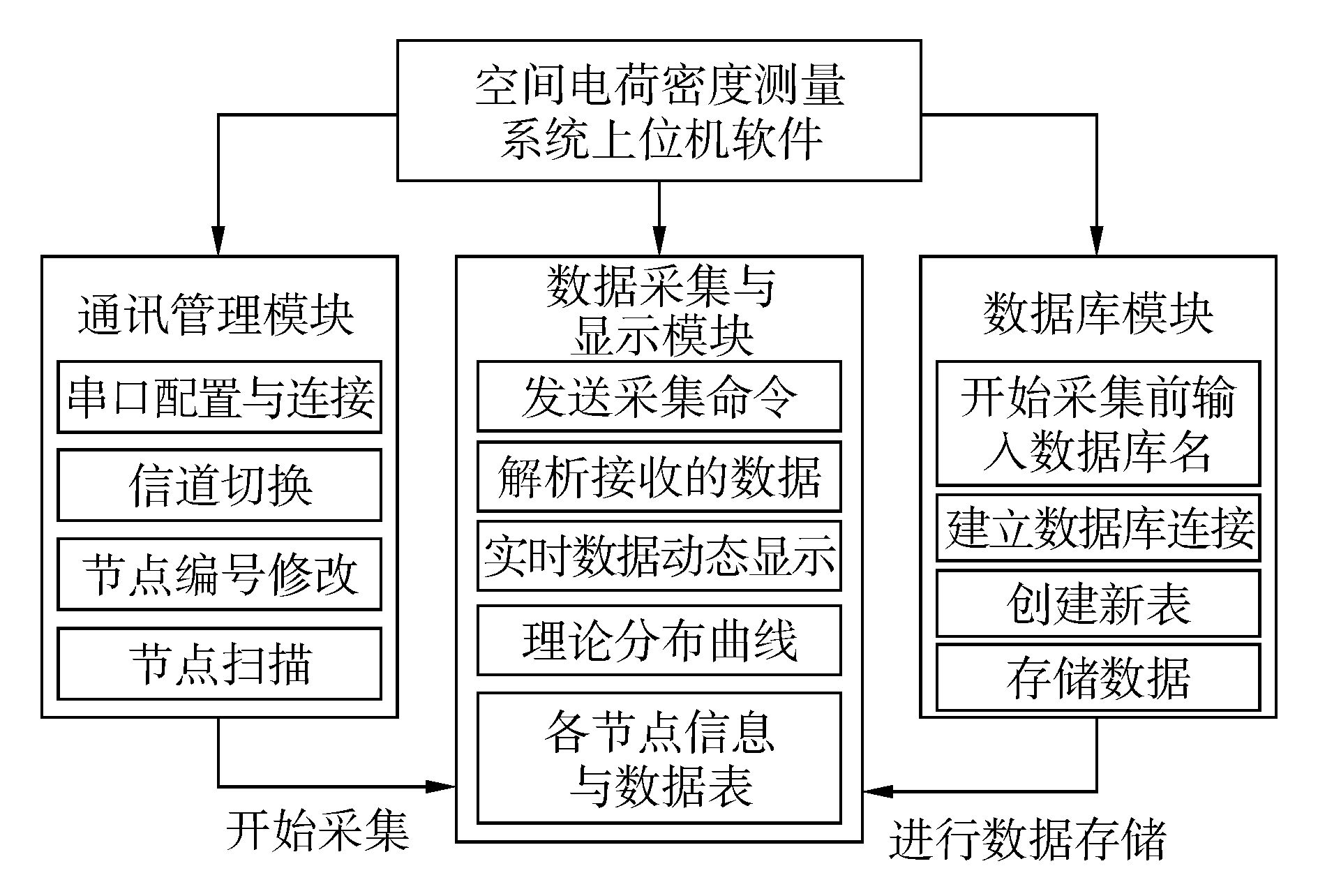图 9 上位机软件的总体设计
3.2.2 通信管理模块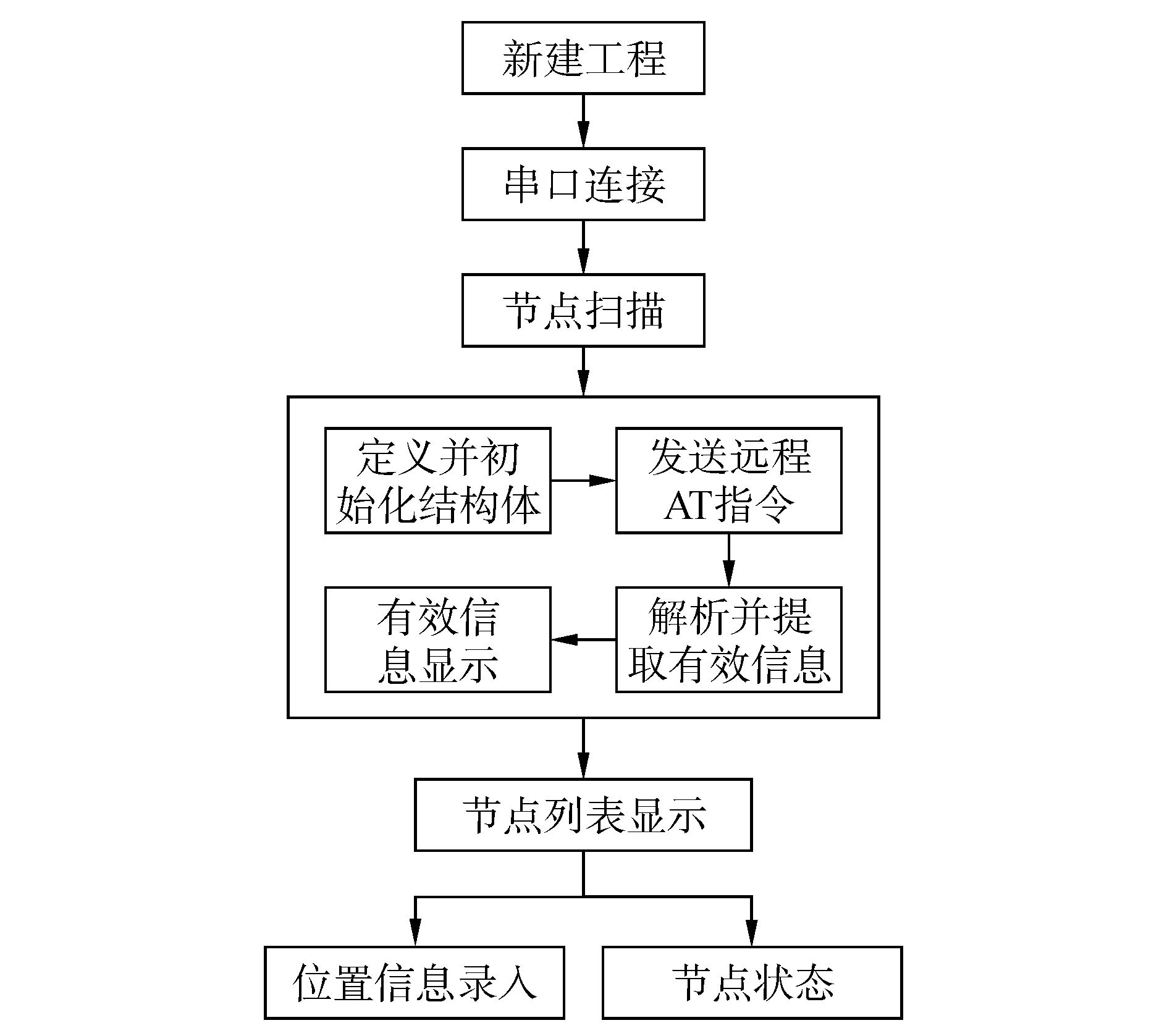图 10 通信管理模块的实现流程

3.2.3 数据采集与显示模块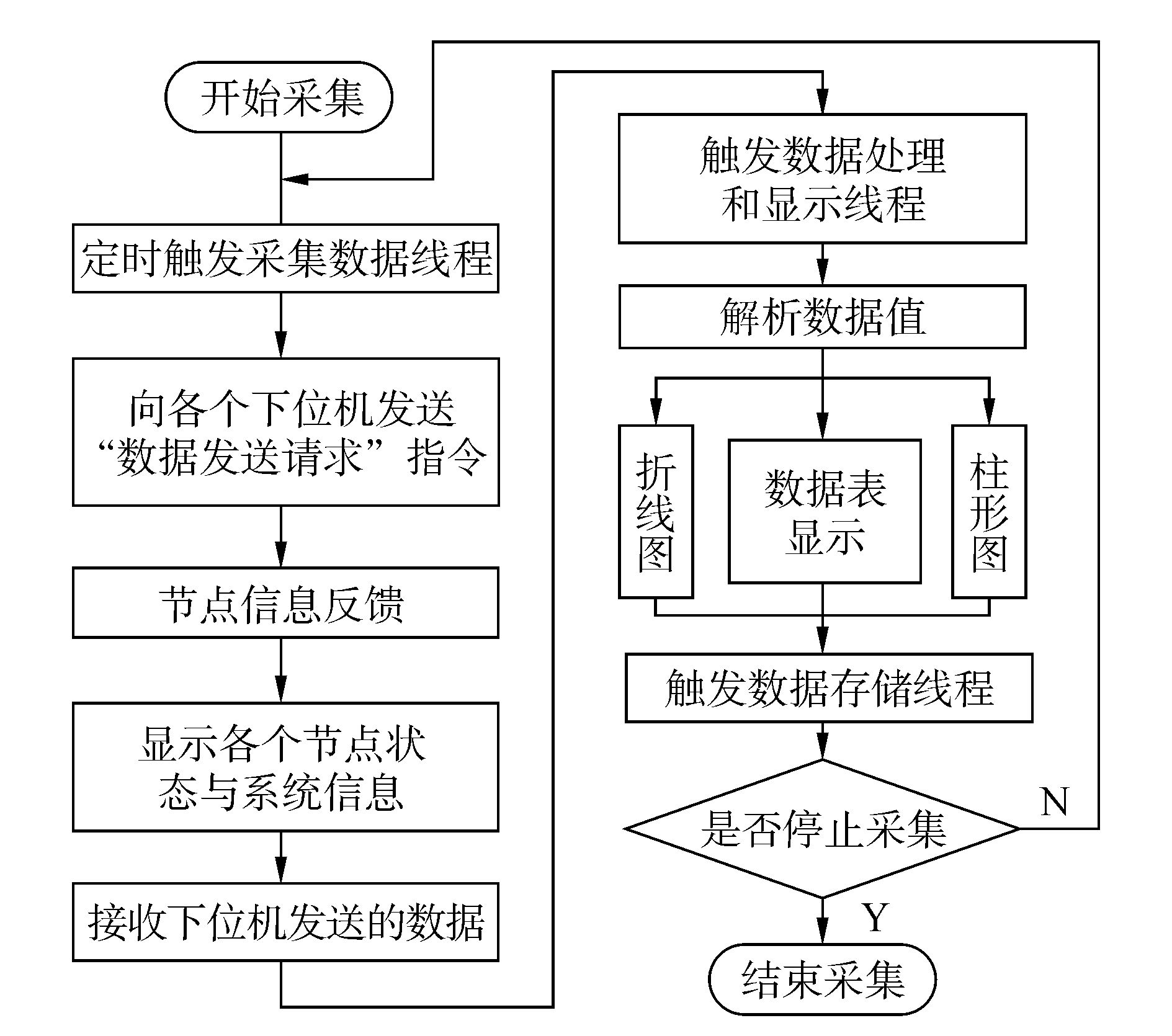图 11 数据采集与显示模块的实现流程
3.2.4 数据存储模块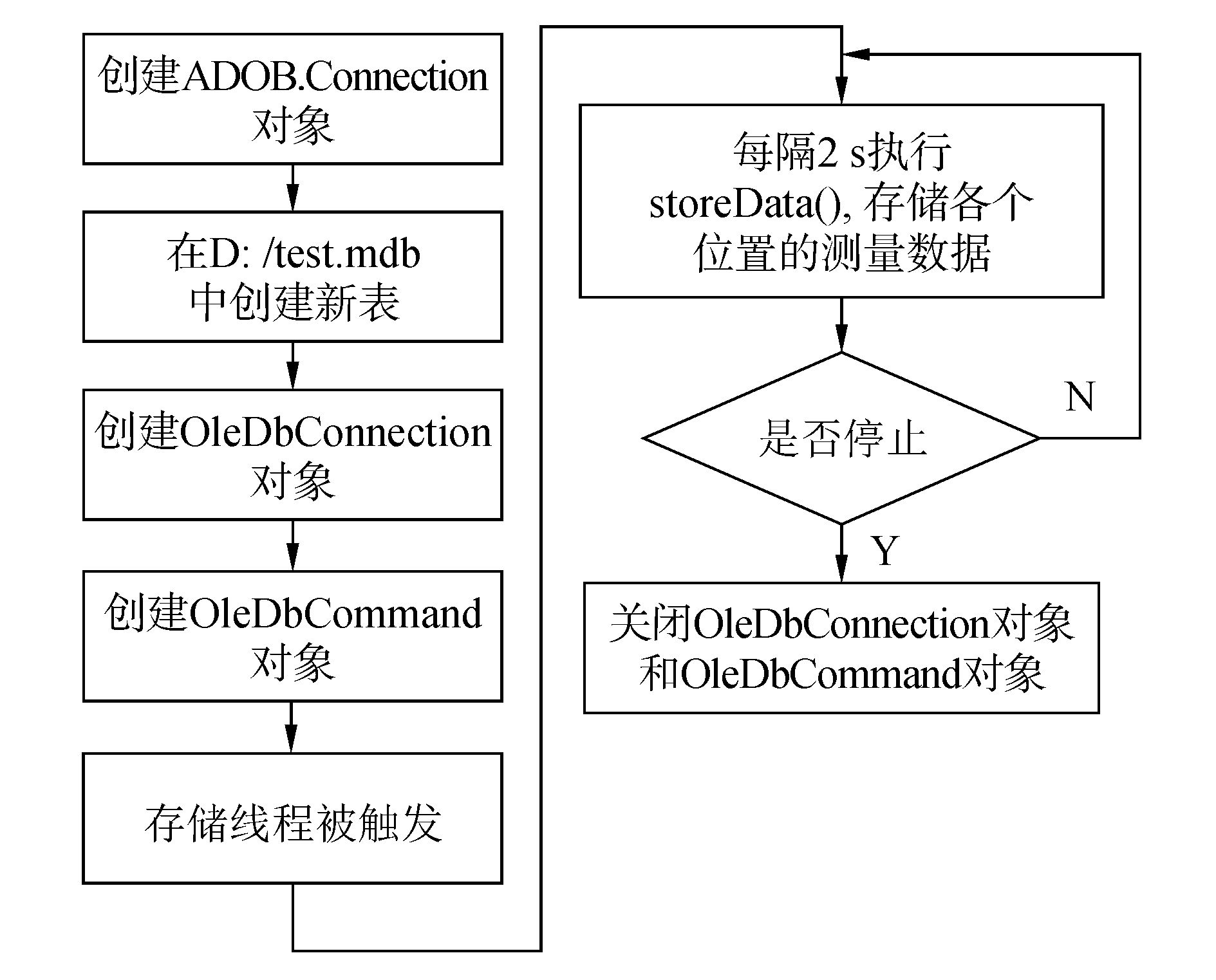图 12 数据存储模块的实现流程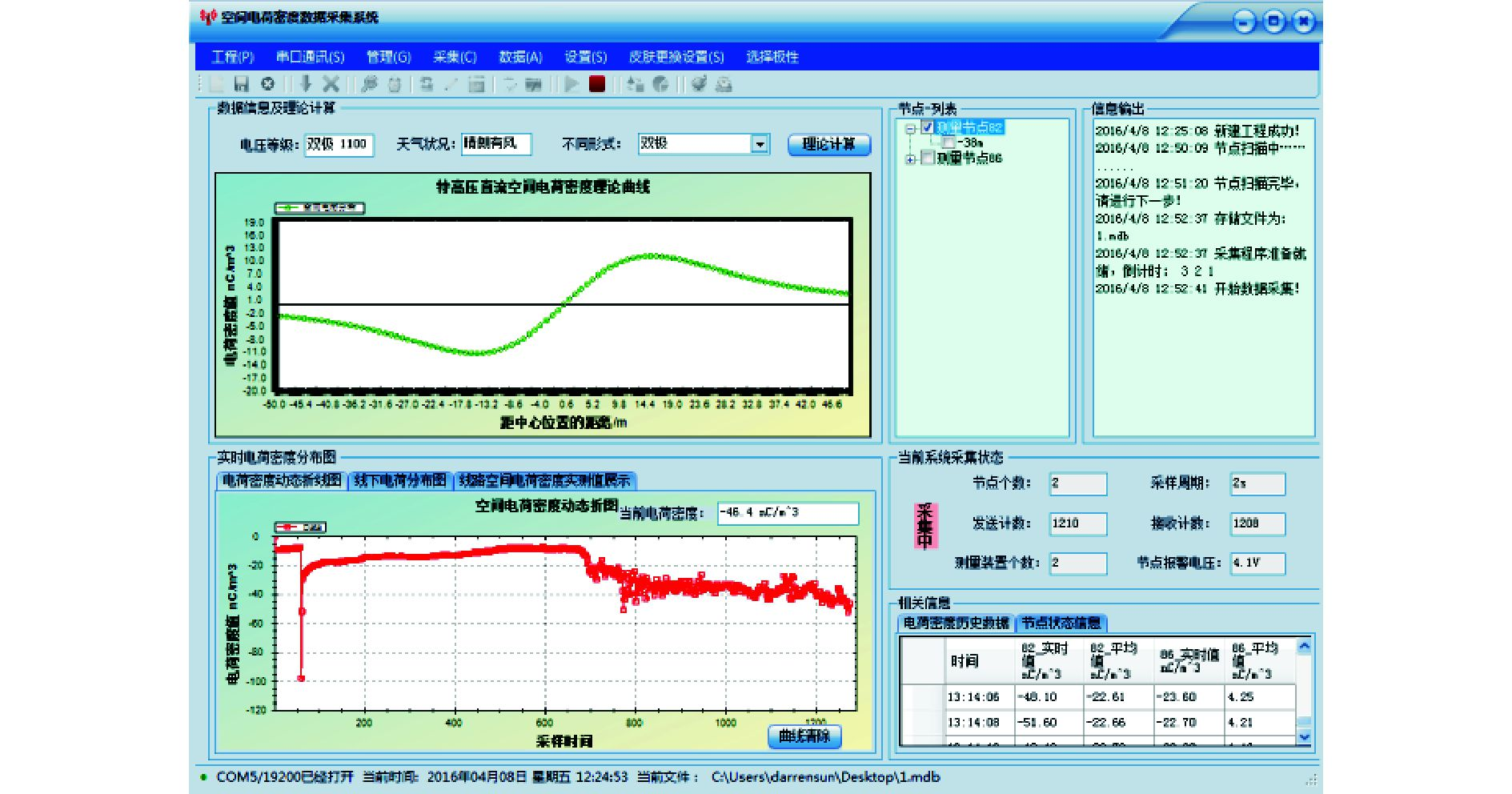图 13 上位机软件主界面
4 现场试验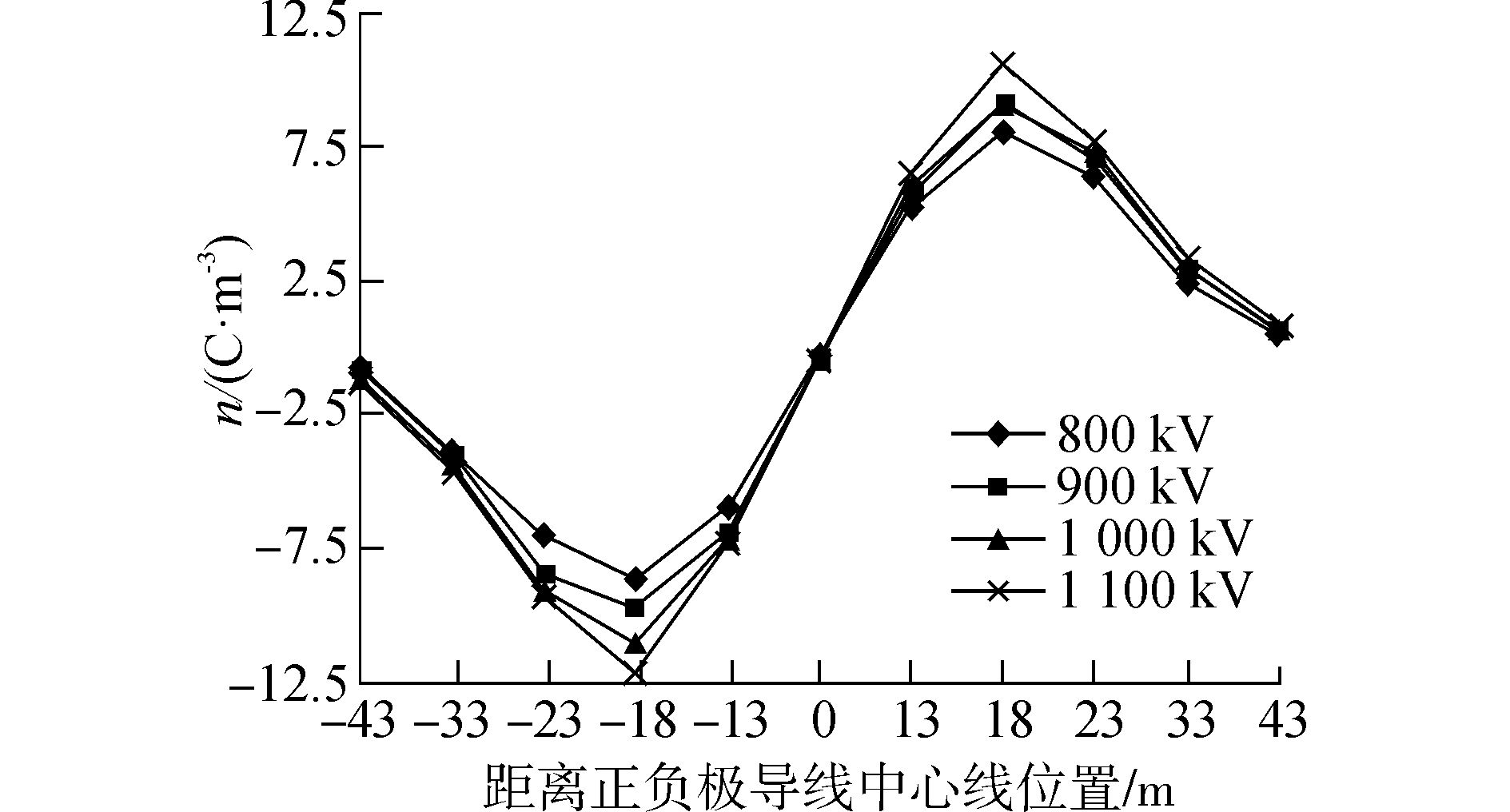图 14 直流线路下空间电荷密度分布情况
5 结论

1) 空间电荷传感器的测量原理为离子计数器法，其结构为同轴圆柱筒型，与以往的平行极板型结构相比有更好的电磁兼容性和气密性。

2) 现场试验结果表明，该测量系统具有极高的可适用性和稳定性。

  麻江帆, 崔勇, 袁海文, 等. 特高压输电线路复杂环境中电场测量系统的电磁兼容设计[J]. 应用科技, 2015, 42(6): 15-19. (0)  周之. 基于声脉冲法的直流输电线路电晕空间电荷测量方法研究[D]. 重庆: 重庆大学, 2013. (0)  RUZEK V, DRINOVSKY J, KEJIK Z. Influence of the probe position to disturbance measurement in the workbench faraday cage[C]//Radioelektronika, 21st International Conference. Brno:IEEE Conference Publications, 2011:1-4. (0)  Product Manual. XBee/XBee-PRO DigiMesh 2.4 RF Modules[EB/OL]. Minnetonka, Minnesota:Digi International Inc[2016-05-24]. http://ftp1.digi.com/support/documentation/90000991_E.pdf. (0)  严守道, 袁海文, 陆家榆, 等. 复杂环境下地面合成电场测量系统的研究[J]. 电网技术, 2013, 37(1): 183-189. (0)  王利恒, 李昌禧. 热式气体流量计温度补偿研究[J]. 传感技术学报, 2008, 21(8): 1379-1382. (0)  梁国伟, 文英杰, 黄震威, 等. 热式气体流量计原理及影响因数分析研究[J]. 中国计量学院学报, 2008, 19(3): 201-205, 224. (0)  葛瑞祎. 特高压直流输电线路下空间电荷分布研究[D]. 北京: 北京航空航天大学, 2014. (0)  倪宁, 肖雪夫, 葛良全, 等. 微弱电流的I-V变换测量方法研究[J]. 核电子学与探测技术, 2013, 33(6): 665-669. (0)  邹岸新. 特高压直流输电线路下离子流场的仿真计算研究[D]. 重庆: 重庆大学, 2012. (0)  张宇, 阮江军. HVDC输电线路离子流场数值计算法方法研究[J]. 高电压技术, 2006, 32(9): 140-142. (0)  王毅, 孙成秋, 汤涛, 等. 不同运行方式下特高压直流输电线路的地面电场与离子流分布[J]. 电网技术, 2008, 32(2): 29-33. (0)